### Home > AC > Chapter 2 > Lesson 2.1.5 > Problem2-53

2-53.

Copy and complete each of the Diamond Problems below. The pattern used in the Diamond Problems is shown at right.1.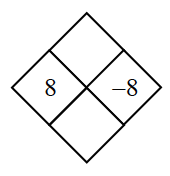Multiply $8$ by $−8$ to get $xy$.
$8(−8)=−64$

Add $8$ to $-8$ to get $x+y$.
$8+(−8)=0$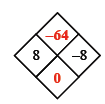1.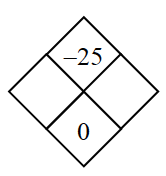What are the factors of $−25$?

Which of these add to $0$?

1.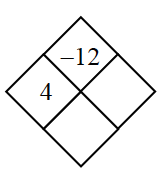Divide $−12$ by $4$ to find $y$.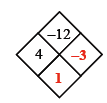1.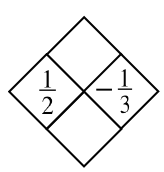Use the pattern in the diamond and remember your negative signs.